# A weight suspended by two strings

LLR

## Homework Statement

A weight is suspended by two strings at two different angles (the picture is included in the attempted solution)
a.) Find the expressions for the tension in each of the strands
b.) Assuming the tension, T1, does not change, what will be the acceleration of the weight if the second string is released?

ΣF = ma

## The Attempt at a Solution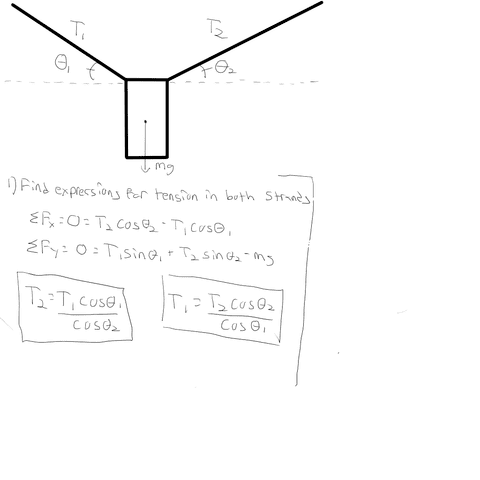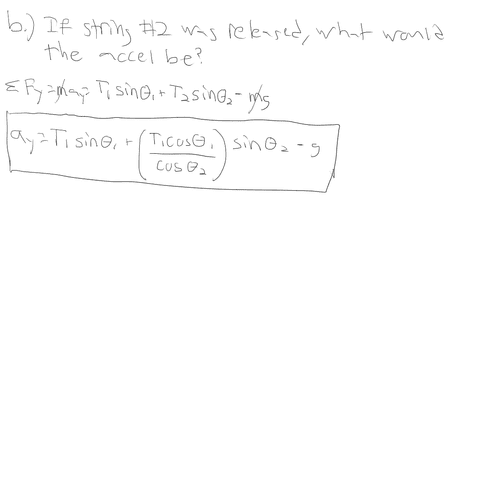This is my attempted solution for the problem. A) I think I'm good with but if there's something wrong please point it out. B) is where I really have trouble. I'm not sure I did accel in the Y correctly (I had substituted the equation for T2 into my B equation, sorry I didn't show that).

I just feel like I'm missing something here, like it should be simpler than that. Also I am unsure if there will be acceleration in the X. But if there was I imagine I would figure it similarly to the way I did Y[/B]

voko
A is not finished. The two expressions that you obtained refer to each circularly.

In B, you have to assume that tension 2 disappears, while tension 1 is entirely present.

LLR
Okay, let me start with A and see if I'm on the right track

Lets say I keep what I got for T2. Would I substitute that in for the second equation and solve for T1? My algebra is admittedly kind of rusty, and I'm having trouble isolating both T1s by themselves when I do that

voko
In A, you had two starting equations. You used one of the to obtain the relationship between tensions. Use the other one to determine the tensions completely.

LLR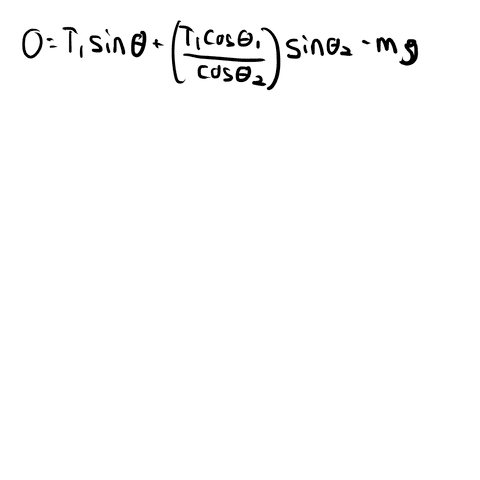Kind of like this? Or am I not doing this correctly?

EDIT: Obviously I didn't solve this yet, but when solved would this be correct? I haven't solved it because I have trouble getting T1 by itself since there is two of them in the equation

Homework Helper
Gold Member
2022 AwardKind of like this? Or am I not doing this correctly?

EDIT: Obviously I didn't solve this yet, but when solved would this be correct? I haven't solved it because I have trouble getting T1 by itself since there is two of them in the equation
Yes. How would you rewrite 5x+3x so that there is only one x?

LLR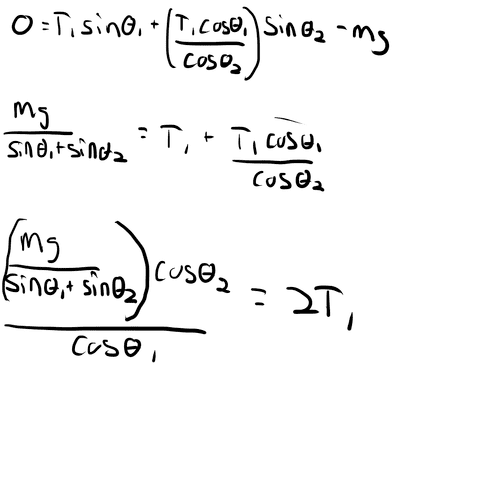This is what I end up getting but it really doesn't seem right. Once again I apologize for my incredibly rusty algebra

voko
No that is not correct. You need to brush up on your algebra.

Homework Helper
Rusty is a good term. Dissolve some of the rust by
* taking one step at the time
* doing on the right the same what you do on the left
* rewriting things like T1 bla + T1 da as T1 (bla + da)
* gradually bringing what you want to know (T1) to one side
so

T1 bla + T1 da - mg = 0
T1 ( bla + da) - mg = 0 ##\ \ \ \ \ ## group terms with T1
T1 ( bla + da) = mg ##\ \ \ \ \ ## add mg to left and right
T1 = mg/( bla + da) ##\ \ \ \ \ ## divide by ( bla + da) left and right (make sure it's never zero)

LLR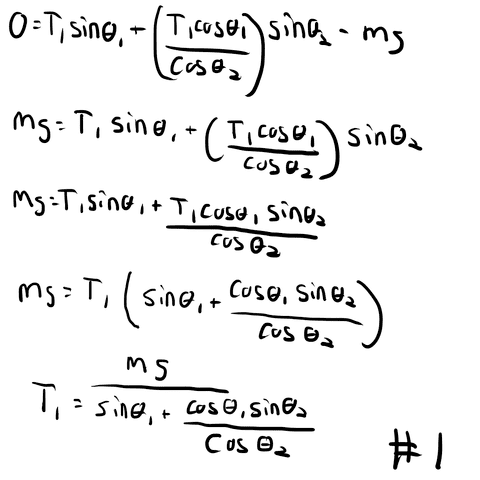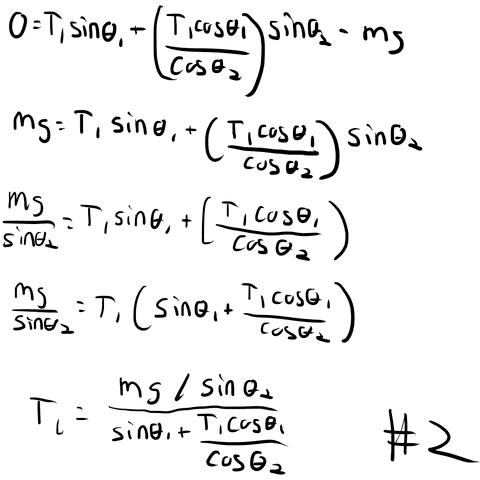Something like this? I have two versions as I was unsure whether to combine the terms or divide the sin

Thank you all for your replies though, it has been a while since any sort of math class and I know I'm still really rough on it

Homework Helper
Version 2 step 2: You should divide both terms on the right by sin theta2

LLR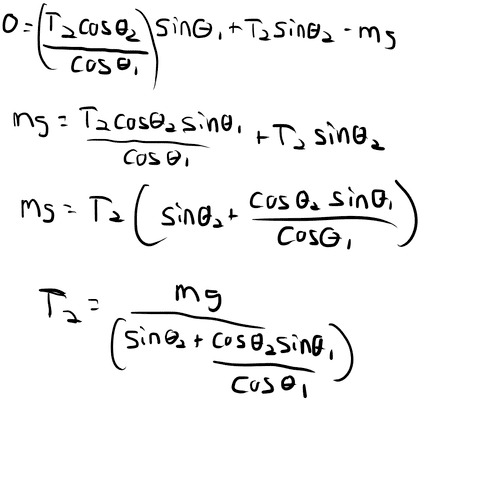Alright, so using the same methods as above I solve for T2 in a similar manner. This should take care of part A, assuming this is correct?

LLR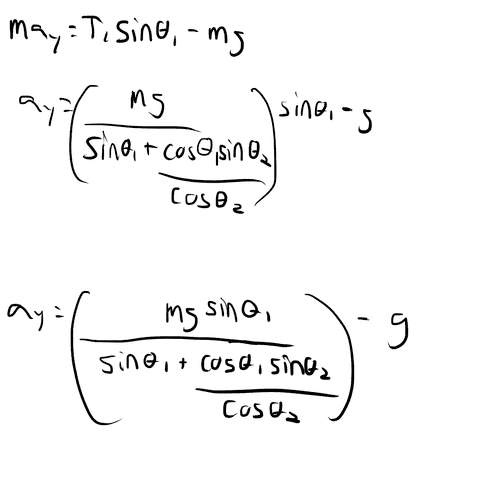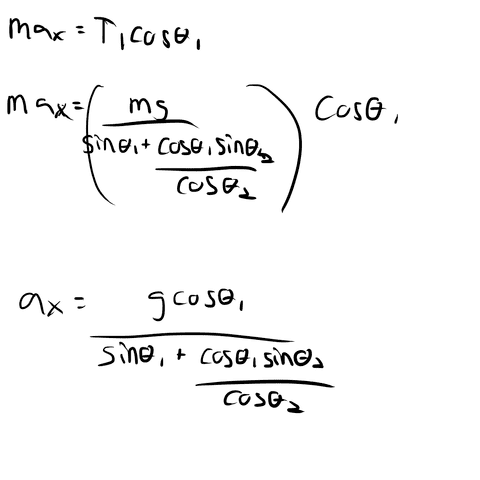I went ahead and plugged in the equations from part A to solve part B, let me know if I messed up somewhere please

Homework Helper
Gold Member
2022 Award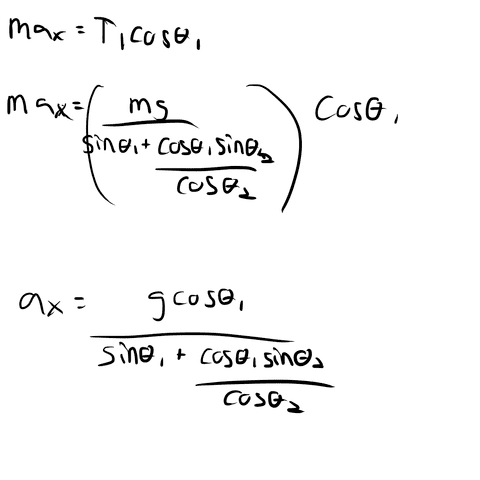I went ahead and plugged in the equations from part A to solve part B, let me know if I messed up somewhere please
In your ay calculation you failed to cancel an m. There should be no mass terms in the answer.
All your answers can be simplified by multiplying top and bottom by cos theta2. It's a bit ugly having a division inside a division.
There is a quicker way to get (b) having got (a). Since T1 doesn't change, the only difference in the system of forces is the removal of T2. This is equivalent, as far as the mass is concerned, to adding a force -T2. So the acceleration is -T2/m.

LLR
So the final answer would look something like this?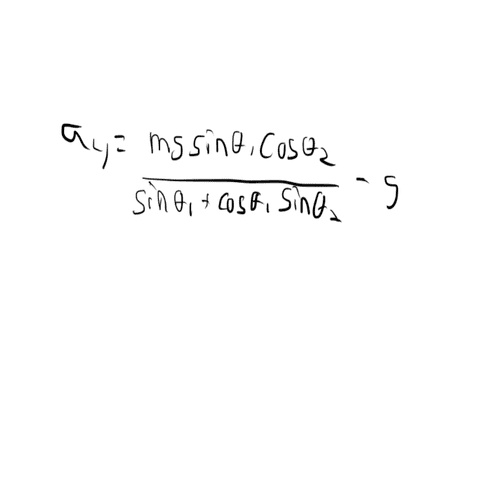Or am I multiplying the top and bottom wrong?

I aplogize I forget to remove the extra m from this one, but I'm a bit confused as to why it would cancel. The starting equation has 1 m on one side, and 2 on the other. Wouldn't only one m on each side cancel then?

And I'm afraid I'm not quite following why it would only be a = -T2/m. Why would T1 not be factored into it?

Homework Helper
Gold Member
2022 Award
So the final answer would look something like this?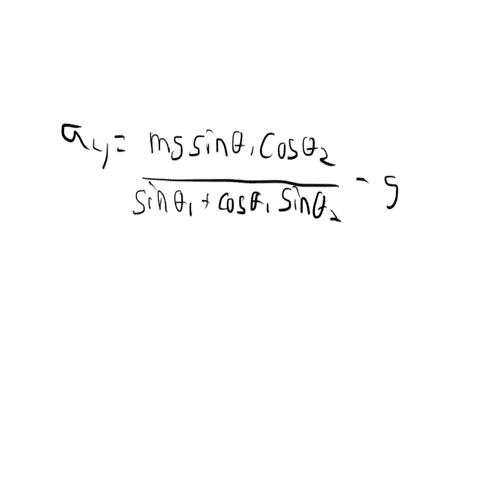Or am I multiplying the top and bottom wrong?

I aplogize I forget to remove the extra m from this one, but I'm a bit confused as to why it would cancel. The starting equation has 1 m on one side, and 2 on the other. Wouldn't only one m on each side cancel then?

And I'm afraid I'm not quite following why it would only be a = -T2/m. Why would T1 not be factored into it?